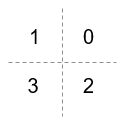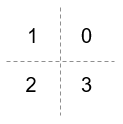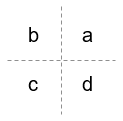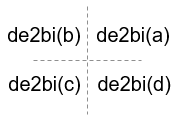Documentation

OQPSK Demodulator Baseband

Demodulation using OQPSK method

• Library:
• Communications Toolbox / Modulation / Digital Baseband Modulation / PMDescription

The OQPSK Demodulator Baseband block applies pulse shape filtering to the input waveform and demodulates it using the offset quadrature phase shift keying (OQPSK) method. For more information, see Pulse Shaping Filter. The input is a baseband representation of the modulated signal.

For information about delays incurred by modulator-demodulator pair processing, see Modulation Delays.

Ports

Input

expand all

Input baseband waveform, specified as a discrete-time complex scalar or column vector.

The block processes the input signal based on the Output type setting.

Data Types: double
Complex Number Support: Yes

Output

expand all

Output data, returned as an integer or bit column vector.

Parameters

expand all

Modulation

Output type, specified as Integer or Bit.

• When you set Output type to Integer, the block outputs a vector of integer symbols with values from 0 to 3, the length of which is the number of output symbols.

• When you set Output type to Bit, the block outputs a 2-bit binary representation of integers, in a binary-valued, even-length vector.

The input period for each integer or bit pair is the Samples per symbol times the output sample period.

Phase of zeroth point of the signal constellation in radians, specified as a scalar.

Example: Setting Phase offset (rad) to 0 aligns the OQPSK signal constellation points on the axes, {(1,0), (0,j), (-1,0), (0,-j)}.

Signal constellation bit mapping, specified as Gray, Binary, or a custom 4-element numeric vector of integers with values from 0 to 3.

SettingConstellation Mapping for IntegersConstellation Mapping for BitsComment

GrayThe signal constellation mapping is Gray-encoded.

BinaryThe signal constellation mapping for the input integer m (0 ≤ m ≤ 3) is the complex value e(j*(PhaseOffset+π/4) + j*2*π*m/4).

Custom 4-element numeric vector of integers with values from 0 to 3Elements [a b c d] must be composed of the set of values [0, 1, 2, 3] in any order.

Filtering

Select the filtering pulse shape: Half sine, Normal raised cosine, Root raised cosine, or Custom.

Raised cosine filter rolloff factor, specified as a scalar from 0 to 1.

Dependencies

This property appears when Pulse shape is Normal raised cosine or Root raised cosine.

Data Types: double

Filter length in symbols, specified as a scalar. An ideal raised cosine filter has an infinite impulse response. However, to realize a practical implementation of this filter, the object truncates the impulse response to Filter span (in symbols) symbols.

Dependencies

This property appears when Pulse shape is Normal raised cosine or Root raised cosine.

Data Types: double

Filter numerator, specified as a row vector.

Dependencies

This parameter appears when Pulse shape is Custom.

Data Types: double

Number of samples per symbol, specified as a positive even integer.

Data Types: double

Other Parameters

Select the output data type: double, single, or uint8.

Block Characteristics

 Data Types Boolean | double | integer | single Multidimensional Signals no Variable-Size Signals no

expand all